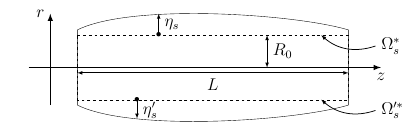# Theory of Solid Mechanics

## 1. Notations and units

 Notation Quantity Unit $\boldsymbol{\eta}_s$ displacement $m$ $\rho_s$ density $kg.m^{-3}$ $\lambda_s$ first Lamé coefficients $N.m^{-2}$ $\mu_s$ second Lamé coefficients $N.m^{-2}$ $E_s$ Young modulus $kg.m^{-1}.s^{-2}$ $\nu_s$ Poisson’s ratio dimensionless $\boldsymbol{F}_s$ deformation gradient $\boldsymbol{\Sigma}_s$ second Piola-Kirchhoff tensor $f_s^t$ body force
• strain tensor $\boldsymbol{F}_s = \boldsymbol{I} + \nabla \boldsymbol{\eta}_s$

• Cauchy-Green tensor $\boldsymbol{C}_s = \boldsymbol{F}_s^{T} \boldsymbol{F}_s$

• Green-Lagrange tensor

\begin{align} \boldsymbol{E}_s &= \frac{1}{2} \left( \boldsymbol{C}_s - \boldsymbol{I} \right) \\ &= \underbrace{\frac{1}{2} \left( \nabla \boldsymbol{\eta}_s + \left(\nabla \boldsymbol{\eta}_s\right)^{T} \right)}_{\boldsymbol{\epsilon}_s} + \underbrace{\frac{1}{2} \left(\left(\nabla \boldsymbol{\eta}_s\right)^{T} \nabla \boldsymbol{\eta}_s \right)}_{\boldsymbol{\gamma}_s} \end{align}

## 2. Equations

Newton’s second law allows us to define the fundamental equation of solid mechanics, as follows

$\rho^*_{s} \frac{\partial^2 \boldsymbol{\eta}_s}{\partial t^2} - \nabla \cdot \left(\boldsymbol{F}_s \boldsymbol{\Sigma}_s\right) = \boldsymbol{f}^t_s$

### 2.1. Linear elasticity

\begin{align} \boldsymbol{F}_s &= \text{Identity} \\ \boldsymbol{\Sigma}_s &=\lambda_s tr( \boldsymbol{\epsilon}_s)\boldsymbol{I} + 2\mu_s\boldsymbol{\epsilon}_s \end{align}

### 2.2. Hyperelasticity

#### 2.2.1. Saint-Venant-Kirchhoff

$\boldsymbol{\Sigma}_s=\lambda_s tr( \boldsymbol{E}_s)\boldsymbol{I} + 2\mu_s\boldsymbol{E}_s$

#### 2.2.2. Neo-Hookean

$\boldsymbol{\Sigma}_s= \mu_s J^{-2/3}(\boldsymbol{I} - \frac{1}{3} \text{tr}(\boldsymbol{C}) \ \boldsymbol{C}^{-1})$
$\boldsymbol{\Sigma}_s^ = \boldsymbol{\Sigma}_s^\text{iso} + \boldsymbol{\Sigma}_s^\text{vol}$
##### Isochoric part : $\boldsymbol{\Sigma}_s^\text{iso}$
Table 1. Isochoric law
Name $\mathcal{W}_S(J_s)$ $\boldsymbol{\Sigma}_s^{\text{iso}}$

Neo-Hookean

$\mu_s J^{-2/3}(\boldsymbol{I} - \frac{1}{3} \text{tr}(\boldsymbol{C}) \ \boldsymbol{C}^{-1})$

##### Volumetric part : $\boldsymbol{\Sigma}_s^\text{vol}$
Table 2. Volumetric law
Name $\mathcal{W}_S(J_s)$ $\boldsymbol{\Sigma}_s^\text{vol}$

classic

$\frac{\kappa}{2} \left( J_s - 1 \right)^2$

simo1985

$\frac{\kappa}{2} \left( ln(J_s) \right)$

### 2.3. Axisymmetric reduced model

Here, we are interested in a 1D reduced model, named generalized string.

The axisymmetric form, which will interest us here, is a tube of length $L$ and radius $R_0$. It is oriented following the $z$ axis and $r$ represents the radial axis. The reduced domain, named $\Omega_s^*$ is represented by the dotted line. So, the radial displacement $\eta_s$ is calculated in the domain $\Omega_s^*=\lbrack0,L\rbrack$.

We introduce then $\Omega_s^{'*}$, where we also need to estimate a radial displacement as before. The unique variance is this displacement direction.Figure 1 : Geometry of the reduced model

The mathematical problem associated to this reduced model can be described as

$\rho^*_s h \frac{\partial^2 \eta_s}{\partial t^2} - k G_s h \frac{\partial^2 \eta_s}{\partial x^2} + \frac{E_s h}{1-\nu_s^2} \frac{\eta_s}{R_0^2} - \gamma_v \frac{\partial^3 \eta}{\partial x^2 \partial t} = f_s.$

where $\eta_s$ is the radial displacement that satisfies this equation, $k$ is the Timoshenko’s correction factor, and $\gamma_v$ is a viscoelasticity parameter. The material is defined by its density $\rho_s^*$, its Young’s modulus $E_s$, its Poisson’s ratio $\nu_s$ and its shear modulus $G_s$

In the end, we take $\eta_s=0\text{ on }\partial\Omega_s^*$ as a boundary condition, which will fix the wall to its extremities.